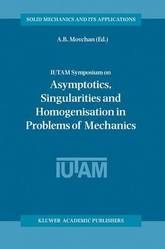Filter
(found 450 products)This volume contains the Proceedings of the IUTAM Symposium held in Liverpool in 2002. It includes the articles presenting the results of recent work in mathematical modelling that covers the following areas of continuum mechanics and theoretical physics: *-Perturbation problems for partial differential equations and their applications in mechanics; * ...
IUTAM Symposium on Asymptotics, Singularities and Homogenisation in Problems of Mechanics
This volume contains the Proceedings of the IUTAM Symposium held in Liverpool in 2002. It includes the articles presenting the results of recent work in mathematical modelling that covers the following areas of continuum mechanics and theoretical physics: *-Perturbation problems for partial differential equations and their applications in mechanics; * Analysis of singular fields; * Homogenisation theory in models of composite structures; * Mathematical models of cracks in solids; * Wave propagation, scattering; * Models of photonic and phononic band gap composite structures; * Advanced numerical techniques.